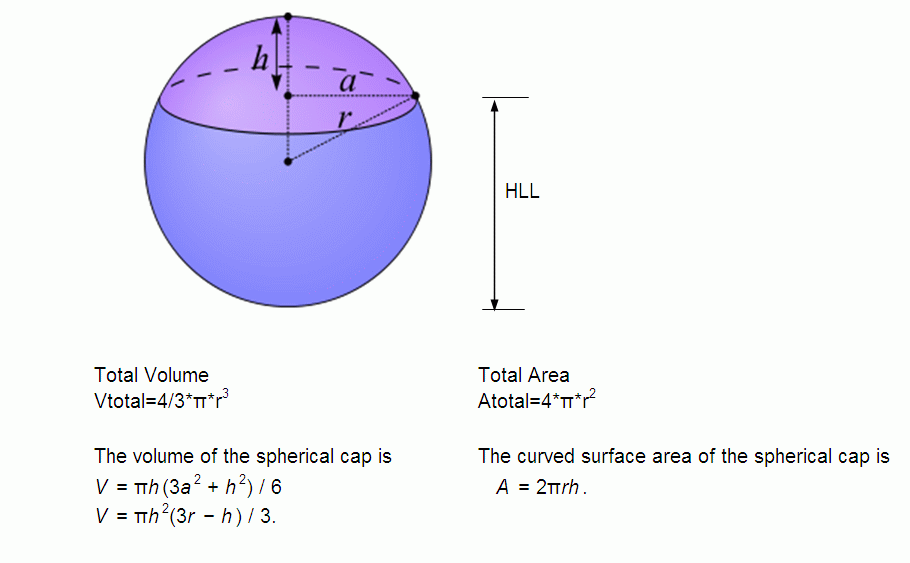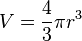# Java Program To Calculate Volume Of Sphere – 3 Simple Ways

Java program to calculate the volume of a sphere. Finding the volume of Sphere is quite simple, if you knew the formula, you can do it. There may be so many ways to represent the code. Below we share the three simple ways to find the volume of a sphere. Check it out.

1. Example # 1 – A simple java program universally applicable.
2. Example # 2 – Using the command line argument # universally applicable.
3. Example # 3 – Using a static method – # Universally applicable.

Also, don’t forget the to  # check out the online compiler and execution tool below #

1. What is a sphere?

A: Around solid figure with every point on its surface is equal distance from its centre. check out the below figure, you will get an idea.imageSource

2) what is the formula to find out the volume of a sphere?

Here is the simple formula to calculate:-Example java program: Example method or sample method #1 :

Output :

Online execution & Compiler Tool here :

Now, if you knew the basic level of programming then no need to check out the explanation of java code here. Just skip the following steps and move on to the next level( example method # 2 ). Here goes the complete explanation of the above code.

– The scanner is an inbuilt package within JDK, can use to read the input values from the user side.

– A Class is a blueprint where objects are created. You can refer here.

– The Scanner can read the input variables from any device ( java.util.scanner ). Whereas system.in can read the input variable from your system or device.

– The output will be displayed here, it’s a su=yntax to represent whatever you were written in those brackets. Remember it has an output method syntax. You can write it as in so many methods, instead of println , you can also use the Format also.

– Double is a datatype, where can store the values. Whereas ‘r’ is a name to the memory that we are using.

– The formula to calculate the volume of a sphere.

– The output will be displayed here.

Also, check :

That’s the complete explanation of the above java program with examples as well. Make sure to use the online compiler and execution tool in order to know how the program works. Below is another sample method or example method to find the volume. Consider it has example method – 2.

Example Method – 2 # command line argument #

Here is another example, there may be no difference while comparing with the above program. Here instead of ” Scanner ” we are using the ” parse “ method.

The main difference is as ” suppose in the least case if the Scanner class file is missing from your JDK file then how can you compile and execute the above program since the scanner can retrieve the data from java.util.Scanner.

Here without that method, you can directly execute the program by using the parse function.

‘ Parse ‘ is a method which can read the strings as argument format like int, float, double. Check out the example method to find out the volume of a sphere.

Online Execution tool for the above code: Enter the values between 1-100 in argument function and click on execute.

Got it right!

Note: It’s all up to you, you can use the whatever datatype that you wish to use. From the above example we are using the ‘ double r =Double.parseDouble(args); or you can use ‘ int r=Int.parseInt(args);.

The difference is nothing but, if you want to display the I/O variables in decimal points then you can use the ‘Double’. Without decimal, use the ‘int’.

Here goes another java program to find the example method. Consider it has another example method #3.

Example method # 3 :

From the above example method #3. Here is the explanation :

Actually, there is no need to represent the program much complicated, as like above ( example 1 and example 2 ) are more than enough. But, in some cases, the question may vary and ask as like ‘ Write a java program to calculate the volume of a sphere using return method ‘.

Here goes the explanation of the above code :

Where ” Return is a keyword which returns the value of an invoked method “.

# Java program to find the volume of a sphere with examples end #